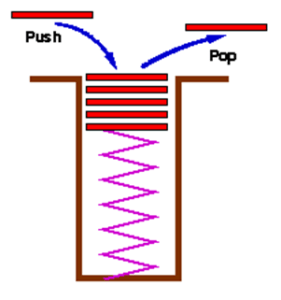# and its application,

A stack is an ordered collection of items into which new items may be inserted and from which items may be deleted at one end called the TOP of the stack

#### What are the properties of these stacks?

You can only add an item (book shirt etc) on top of the stack and you can only remove it from the top too

## BASIC OPERATIONS WITH STACKS

Push🞫 need to check overflow

Pop

🞤 Remove an item

🞫 need to check underflow

stackTop

🞤 What’s on the Top

🞫 Could be empty

## Push

• Adds a new data element to the top of the stack

## Pop

• Removes a data element from the top of the stack

## StackTop

• Checks the top Stack is not changed

• Stacks in real life: stack of books, stack of plates
• Add new items at the top
• Remove an item at the top
• Stack data structure similar to real life: collection of elements arranged in a linear order.
• Can only access element at the top
• A stack is an ordered collection of items into which new items may be inserted and from which items may be deleted at one end only, called top of the stack. i.e. deletion/insertion can only be done from top of the stack
• The insert operation on a stack is often called Push operation and delete operation is called Pop operation.

## OPERATION ON STACK

A Stack should have the following methods

• push(item)    //Push an item onto the stack
• pop( )          //Pop an item off the stack
• isEmpty()  //Return true if stack is empty.
• Isfull( )         //Return true if stack is full

Basic operation code

# Stack by using an Array

#include “stdafx.h”
#include<iostream>
#include<conio.h>
#include<stdlib.h>
using namespace std;
class stack
{
int stk;
int top;
public:
stack()
{
top = -1;
}
void push(int x)
{
if (top > 4)
{
cout << “stack over flow”;
return;
}
stk[++top] = x;
cout << “inserted” << x << endl;
}
void pop()
{
if (top <0)
{
cout << “stack under flow”;
return;
}
cout << “deleted” << stk[top–];
}
void display()
{
if (top<0)
{
cout << ” stack empty”;
return;
}
for (int i = top; i >= 0; i–)
cout << stk[i] << ” “;
}
};

int main()
{
int ch;
stack st;
while (1)
{
cout << “\n1.push \n2.pop \n3.display \n4.exit\n\nEnter ur choice”;
cin >> ch;
switch (ch)
{
case 1: cout << “enter the element”;
cin >> ch;
st.push(ch);
break;
case 2: st.pop(); break;
case 3: st.display(); break;
case 4: exit(0);
}
}
return (0);

}

#include “stdafx.h”
#include <iostream>
#include <stdio.h>
#include <conio.h>
using namespace std;
struct node
{
int data;
node *next;
}*p = NULL, *r = NULL, *q = NULL, *t = NULL;
void push(int x)
{
t = new node;
t->data = x;
t->next = NULL;
if (p == NULL)
{
p = t;

}
else
{
t->next = p;
p = t;

}
}
int pop()
{
int x;
if (p == NULL)
{
cout << “emqty queue\n”;
}
else
{
q = p;
x = q->data;
p = p->next;
delete(q);
return(x);
}
}
int main()
{
/*int n, c = 0, x;
cout << “Enter the number of values to be qushed into stack\n”;
cin >> n;
while (c < n)
{
cout << “Enter the value to be entered into queue = “;
cin >> x;
push(x);
c++;
}
cout << “\n\nRemoved Values\n\n”;
while (true)
{
if (p != NULL)
cout << remove() << endl;
else
break;
}

//write system pause command here

int ch;
while (1)
{
cout << “\n1.push \n2.pop \n3.exit\n\nEnter ur choice”;
cin >> ch;
switch (ch)
{
case 1: cout << “enter the element”;
cin >> ch;
push(ch);
break;
case 2: pop(); break;

case 3: exit(0);
}
}
return (0);
}

• How to access the top of the stack
• How to push data onto the stack
• How to pop data from the stack